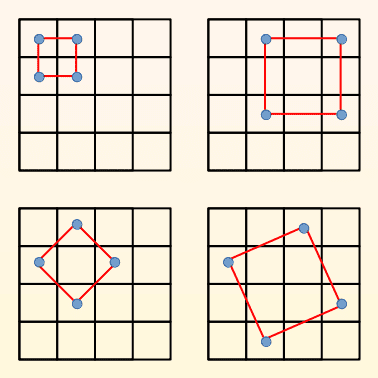# Square Tic Tac Toe GUI

In the previous section you saw how to create a Tic Tac Toe GUI. In this section you'll see how to tweak that code to create a game with different rules. Instead of a line, a square should be formed using four corners.

## How it differs from Tic Tac Toe

In Tic Tac Toe, a player wins by forming a line with three consecutive cells in any direction — horizontal, vertical or diagonal. In this modified version, a player has to form a square, i.e. four cells forming 90 degree angles and equidistant from each other.

A 3x3 grid would be too small a playing area, so 4x4 grid is used instead. Compared to 8 possible lines in Tic Tac Toe, this version has 20 possible squares. Can you spot all of them? Here's an illustration to help you:## Code

``````# square_tic_tac_toe.py
import random
import tkinter as tk

class Root(tk.Tk):
def __init__(self):
super().__init__()

self.title('Square Tic Tac Toe')
self.geometry('500x400')

self.char_x = tk.PhotoImage(file='./char_x.png')
self.char_o = tk.PhotoImage(file='./char_o.png')
self.empty = tk.PhotoImage()

self.active = 'GAME ACTIVE'
self.total_cells = 16
self.corners = 4
self.computer = {'value': 1, 'bg': 'orange',
'win': 'COMPUTER WINS', 'image': self.char_x}
self.user = {'value': self.corners+1, 'bg': 'grey',
'win': 'USER WINS', 'image': self.char_o}
self.board_bg = 'white'
self.all_squares = ((0, 1, 4, 5), (1, 2, 5, 6), (2, 3, 6, 7),
(4, 5, 8, 9), (5, 6, 9, 10), (6, 7, 10, 11),
(8, 9, 12, 13), (9, 10, 13, 14), (10, 11, 14, 15),
(0, 2, 8, 10), (1, 3, 9, 11), (4, 6, 12, 14),
(5, 7, 13, 15), (0, 3, 12, 15), (1, 4, 6, 9),
(2, 5, 7, 10), (5, 8, 10, 13), (6, 9, 11, 14),
(1, 7, 8, 14), (2, 4, 11, 13))

self.create_control_frame()

).pack(side=tk.LEFT)
).pack(side=tk.RIGHT)

def create_control_frame(self):
self.control_frame = tk.Frame()

self.b_quit = tk.Button(self.control_frame, text='Quit',
command=self.quit)
self.b_quit.pack(side=tk.LEFT)

self.b_play = tk.Button(self.control_frame, text='Play',
command=self.play)
self.b_play.pack(side=tk.RIGHT)

def create_status_frame(self):
self.status_frame = tk.Frame()
self.status_frame.pack(expand=True)

tk.Label(self.status_frame, text='Status: ').pack(side=tk.LEFT)
self.l_status = tk.Label(self.status_frame)
self.l_status.pack(side=tk.RIGHT)

def create_board_frame(self):
self.board_frame = tk.Frame()
self.board_frame.pack(expand=True)

self.cell = [None] * self.total_cells
self.board =  * self.total_cells
self.remaining_moves = list(range(self.total_cells))
for i in range(self.total_cells):
self.cell[i] = tk.Label(self.board_frame, highlightthickness=1,
width=60, height=60, bg=self.board_bg,
image=self.empty)
self.cell[i].bind('<Button-1>',
lambda e, move=i: self.user_click(e, move))
r, c = divmod(i, self.corners)
self.cell[i].grid(row=r, column=c)

def play(self):
self.b_play['state'] = 'disabled'
if self.b_play['text'] == 'Play':
self.create_status_frame()
self.b_play['text'] = 'Play Again'
else:
self.board_frame.destroy()
self.l_status['text'] = self.active
self.state = self.active
self.last_click = 0
self.create_board_frame()
self.computer_click()

def quit(self):
self.destroy()

def user_click(self, e, user_move):
if self.board[user_move] != 0 or self.state != self.active:
return
self.update_board(self.user, user_move)
if self.state == self.active:
self.computer_click()

def computer_click(self):
computer_move = random.choice(self.remaining_moves)
self.update_board(self.computer, computer_move)

def update_board(self, player, move):
self.board[move] = player['value']
self.remaining_moves.remove(move)
self.cell[self.last_click]['bg'] = self.board_bg
self.last_click = move
self.cell[move]['image'] = player['image']
self.cell[move]['bg'] = player['bg']
self.update_status(player)
self.l_status['text'] = self.state
if self.state != self.active:
self.b_play['state'] = 'normal'

def update_status(self, player):
winner_sum = self.corners * player['value']
for square in self.all_squares:
if sum(self.board[i] for i in square) == winner_sum:
self.state = player['win']
self.highlight_winning_squares(player, square)
if self.state == self.active and not self.remaining_moves:
self.state = 'TIE'

def highlight_winning_squares(self, player, square):
for i in square:
self.cell[i]['bg'] = player['bg']

if __name__ == '__main__':
root = Root()
root.mainloop()
``````

## Code diff and explanation

The main changes required are board dimensions and indexes of all valid squares. Here's a list of all the changes:

• GUI title changed from `Tic Tac Toe` to `Square Tic Tac Toe`
• `total_cells` changed from `9` to `16`
• Name changed from `line_size` to `corners` and value changed from `3` to `4`
• Name changed from `line` to `square`
• Name changed from `highlight_winning_line` to `highlight_winning_squares`
• `width` and `height` changed from `75` to `60` (you could also increase the GUI window size instead)
• Name changed from `all_lines` to `all_squares` and the new valid indexes populated for 20 possible squares

## Screenshots